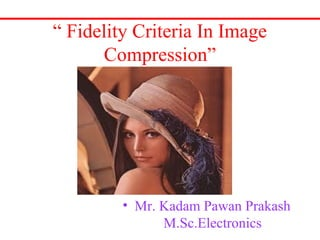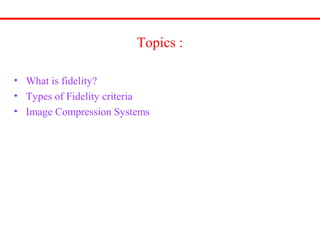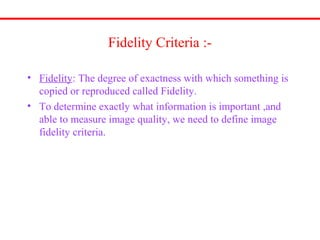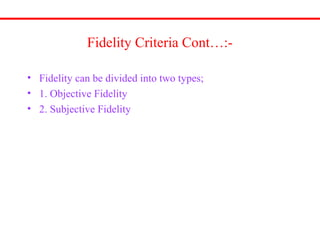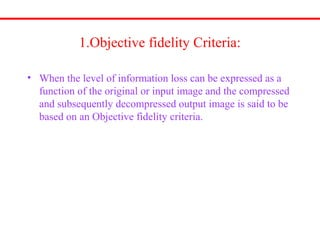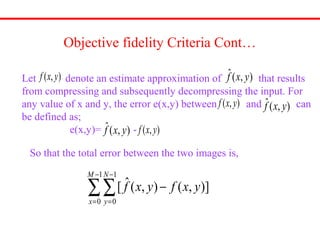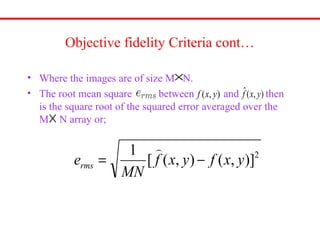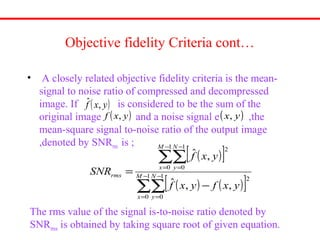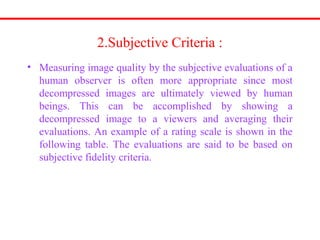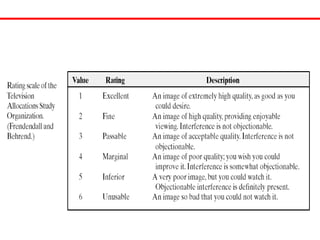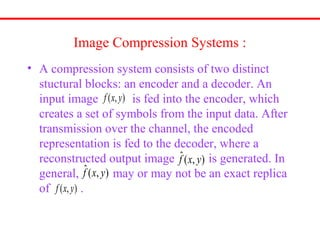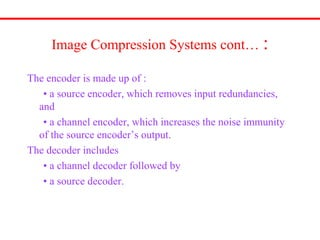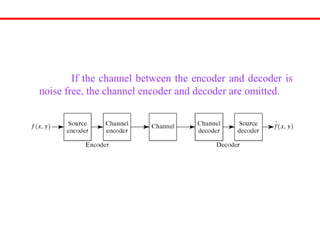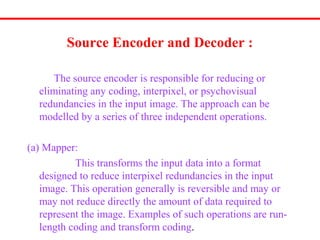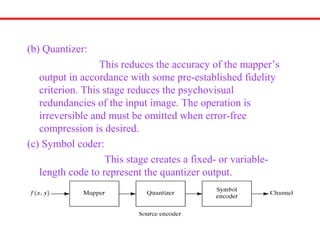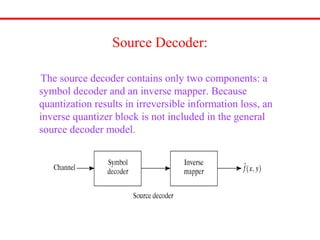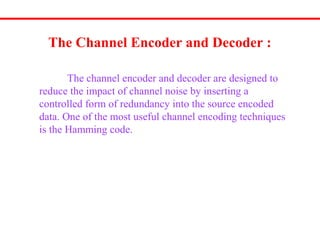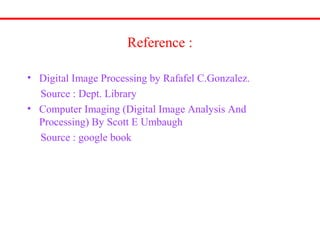1 of 19

### Fidelity criteria in image compression

1. “ Fidelity Criteria In Image Compression” • Mr. Kadam Pawan Prakash M.Sc.Electronics
2. Topics : • What is fidelity? • Types of Fidelity criteria • Image Compression Systems
3. Fidelity Criteria :- • Fidelity: The degree of exactness with which something is copied or reproduced called Fidelity. • To determine exactly what information is important ,and able to measure image quality, we need to define image fidelity criteria.
4. Fidelity Criteria Cont…:- • Fidelity can be divided into two types; • 1. Objective Fidelity • 2. Subjective Fidelity
5. 1.Objective fidelity Criteria: • When the level of information loss can be expressed as a function of the original or input image and the compressed and subsequently decompressed output image is said to be based on an Objective fidelity criteria.
6. Objective fidelity Criteria Cont… ),( yxf ),(ˆ yxf Let denote an estimate approximation of that results from compressing and subsequently decompressing the input. For any value of x and y, the error e(x,y) between and can be defined as; e(x,y)= - ),(ˆ yxf),( yxf ),( yxf ),(ˆ yxf So that the total error between the two images is, ]),(),(ˆ[ 1 0 1 0 ∑∑ − = − = − M x N y yxfyxf
7. Objective fidelity Criteria cont… • Where the images are of size M N. • The root mean square between and then is the square root of the squared error averaged over the M N array or; × ),( yxf ),(ˆ yxf × 2 )],(),([ 1 yxfyxf MN erms −= 
8. Objective fidelity Criteria cont… • A closely related objective fidelity criteria is the mean- signal to noise ratio of compressed and decompressed image. If is considered to be the sum of the original image and a noise signal e ,the mean-square signal to-noise ratio of the output image ,denoted by SNRrms is ; ( )[ ] ( ) ( )[ ]∑∑ ∑∑ − = − = − = − = − = 1 0 1 0 2 1 0 1 0 2 ,,ˆ ,ˆ M x N y M x N y rms yxfyxf yxf SNR ( )yxf ,ˆ ( )yxf , ( )yx, The rms value of the signal is-to-noise ratio denoted by SNRms is obtained by taking square root of given equation.
9. 2.Subjective Criteria : • Measuring image quality by the subjective evaluations of a human observer is often more appropriate since most decompressed images are ultimately viewed by human beings. This can be accomplished by showing a decompressed image to a viewers and averaging their evaluations. An example of a rating scale is shown in the following table. The evaluations are said to be based on subjective fidelity criteria.
10. Image Compression Systems : • A compression system consists of two distinct stuctural blocks: an encoder and a decoder. An input image is fed into the encoder, which creates a set of symbols from the input data. After transmission over the channel, the encoded representation is fed to the decoder, where a reconstructed output image is generated. In general, may or may not be an exact replica of . ),( yxf ),( yxf ),(ˆ yxf ),(ˆ yxf
11. Image Compression Systems cont… : The encoder is made up of : • a source encoder, which removes input redundancies, and • a channel encoder, which increases the noise immunity of the source encoder’s output. The decoder includes • a channel decoder followed by • a source decoder.
12. If the channel between the encoder and decoder is noise free, the channel encoder and decoder are omitted.
13. Source Encoder and Decoder : The source encoder is responsible for reducing or eliminating any coding, interpixel, or psychovisual redundancies in the input image. The approach can be modelled by a series of three independent operations. (a) Mapper: This transforms the input data into a format designed to reduce interpixel redundancies in the input image. This operation generally is reversible and may or may not reduce directly the amount of data required to represent the image. Examples of such operations are run- length coding and transform coding.
14. (b) Quantizer: This reduces the accuracy of the mapper’s output in accordance with some pre-established fidelity criterion. This stage reduces the psychovisual redundancies of the input image. The operation is irreversible and must be omitted when error-free compression is desired. (c) Symbol coder: This stage creates a fixed- or variable- length code to represent the quantizer output.
15. Source Decoder: The source decoder contains only two components: a symbol decoder and an inverse mapper. Because quantization results in irreversible information loss, an inverse quantizer block is not included in the general source decoder model.
16. The Channel Encoder and Decoder : The channel encoder and decoder are designed to reduce the impact of channel noise by inserting a controlled form of redundancy into the source encoded data. One of the most useful channel encoding techniques is the Hamming code.
17. Reference : • Digital Image Processing by Rafafel C.Gonzalez. Source : Dept. Library • Computer Imaging (Digital Image Analysis And Processing) By Scott E Umbaugh Source : google book
18. Thanks…!!!!! • Take it easy…